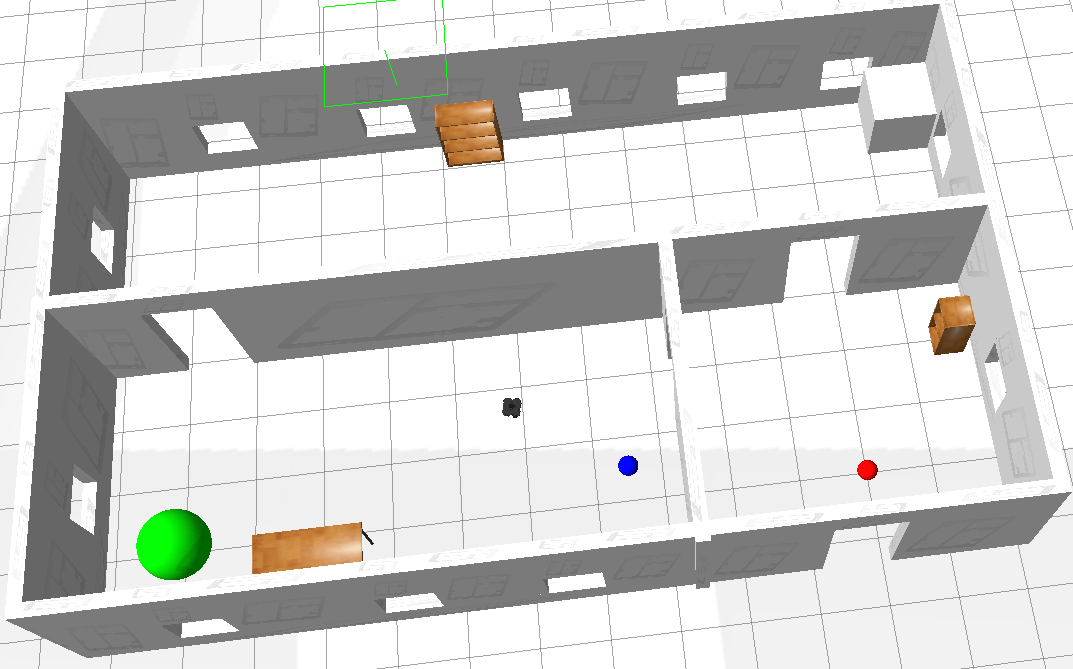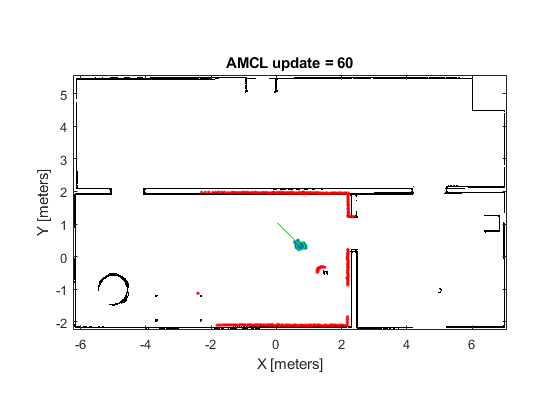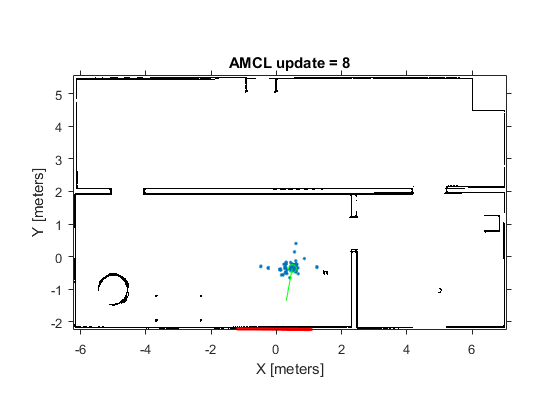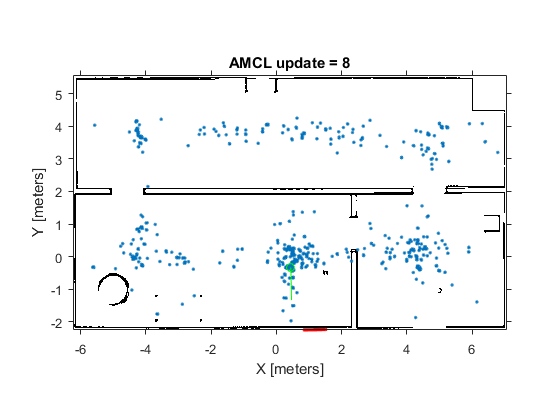# Localize TurtleBot Using Monte Carlo Localization

This example demonstrates an application of the Monte Carlo Localization (MCL) algorithm on TurtleBot® in simulated Gazebo® environment.

Monte Carlo Localization (MCL) is an algorithm to localize a robot using a particle filter. The algorithm requires a known map and the task is to estimate the pose (position and orientation) of the robot within the map based on the motion and sensing of the robot. The algorithm starts with an initial belief of the robot pose's probability distribution, which is represented by particles distributed according to such belief. These particles are propagated following the robot's motion model each time the robot's pose changes. Upon receiving new sensor readings, each particle will evaluate its accuracy by checking how likely it would receive such sensor readings at its current pose. Next the algorithm will redistribute (resample) particles to bias particles that are more accurate. Keep iterating these moving, sensing and resampling steps, and all particles should converge to a single cluster near the true pose of robot if localization is successful.

Adaptive Monte Carlo Localization (AMCL) is the variant of MCL implemented in `monteCarloLocalization`. AMCL dynamically adjusts the number of particles based on KL-distance  to ensure that the particle distribution converge to the true distribution of robot state based on all past sensor and motion measurements with high probability.

The current MATLAB® AMCL implementation can be applied to any differential drive robot equipped with a range finder.

The Gazebo TurtleBot simulation must be running for this example to work.

Prerequisites: Get Started with Gazebo and a Simulated TurtleBot (ROS Toolbox), Access the tf Transformation Tree in ROS (ROS Toolbox), Exchange Data with ROS Publishers and Subscribers (ROS Toolbox).

Note: Starting in R2016b, instead of using the step method to perform the operation defined by the System object, you can call the object with arguments, as if it were a function. For example, `y = step(obj,x)` and `y = obj(x)` perform equivalent operations.

### Connect to the TurtleBot in Gazebo

First, spawn a simulated TurtleBot inside an office environment in a virtual machine by following steps in the Get Started with Gazebo and a Simulated TurtleBot (ROS Toolbox) to launch the `Gazebo TurtleBot World` from the desktop.

In your MATLAB instance on the host computer, run the following commands to initialize ROS global node in MATLAB and connect to the ROS master in the virtual machine through its IP address `ipaddress`. Replace `ipaddress` with the IP address of your TurtleBot in virtual machine.

```ipaddress = '192.168.233.133'; rosinit(ipaddress,11311);```
```Initializing global node /matlab_global_node_26847 with NodeURI http://192.168.233.1:64164/ ```

The layout of simulated office environment:### Load the Map of the Simulation World

Load a binary occupancy grid of the office environment in Gazebo. The map is generated by driving TurtleBot inside the office environment. The map is constructed using range-bearing readings from Kinect® and ground truth poses from `gazebo/model_states` topic.

```load officemap.mat show(map)```### Setup the Laser Sensor Model and TurtleBot Motion Model

TurtleBot can be modeled as a differential drive robot and its motion can be estimated using odometry data. The `Noise` property defines the uncertainty in robot's rotational and linear motion. Increasing the `odometryModel.Noise` property will allow more spread when propagating particles using odometry measurements. Refer to `odometryMotionModel` for property details.

```odometryModel = odometryMotionModel; odometryModel.Noise = [0.2 0.2 0.2 0.2];```

The sensor on TurtleBot is a simulated range finder converted from Kinect readings. The likelihood field method is used to compute the probability of perceiving a set of measurements by comparing the end points of the range finder measurements to the occupancy map. If the end points match the occupied points in occupancy map, the probability of perceiving such measurements is high. The sensor model should be tuned to match the actual sensor property to achieve better test results. The property `SensorLimits` defines the minimum and maximum range of sensor readings. The property `Map` defines the occupancy map used for computing likelihood field. Please refer to `likelihoodFieldSensorModel` for property details.

```rangeFinderModel = likelihoodFieldSensorModel; rangeFinderModel.SensorLimits = [0.45 8]; rangeFinderModel.Map = map;```

Set `rangeFinderModel.SensorPose` to the coordinate transform of the fixed camera with respect to the robot base. This is used to transform the laser readings from camera frame to the base frame of TurtleBot. Please refer to Access the tf Transformation Tree in ROS (ROS Toolbox) for details on coordinate transformations.

Note that currently `SensorModel` is only compatible with sensors that are fixed on the robot's frame, which means the sensor transform is constant.

```% Query the Transformation Tree (tf tree) in ROS. tftree = rostf; waitForTransform(tftree,'/base_link','/camera_depth_frame'); sensorTransform = getTransform(tftree,'/base_link', '/camera_depth_frame'); % Get the euler rotation angles. laserQuat = [sensorTransform.Transform.Rotation.W sensorTransform.Transform.Rotation.X ... sensorTransform.Transform.Rotation.Y sensorTransform.Transform.Rotation.Z]; laserRotation = quat2eul(laserQuat, 'ZYX'); % Setup the |SensorPose|, which includes the translation along base_link's % +X, +Y direction in meters and rotation angle along base_link's +Z axis % in radians. rangeFinderModel.SensorPose = ... [sensorTransform.Transform.Translation.X sensorTransform.Transform.Translation.Y laserRotation(1)];```

### Receiving Sensor Measurements and Sending Velocity Commands

Create ROS subscribers for retrieving sensor and odometry measurements from TurtleBot.

```laserSub = rossubscriber('/scan'); odomSub = rossubscriber('/odom');```

Create ROS publisher for sending out velocity commands to TurtleBot. TurtleBot subscribes to `'/mobile_base/commands/velocity'` for velocity commands.

```[velPub,velMsg] = ... rospublisher('/mobile_base/commands/velocity','geometry_msgs/Twist');```

### Initialize AMCL Object

Instantiate an AMCL object `amcl`. See `monteCarloLocalization` for more information on the class.

```amcl = monteCarloLocalization; amcl.UseLidarScan = true;```

Assign the `MotionModel` and `SensorModel` properties in the `amcl` object.

```amcl.MotionModel = odometryModel; amcl.SensorModel = rangeFinderModel;```

The particle filter only updates the particles when the robot's movement exceeds the `UpdateThresholds`, which defines minimum displacement in [x, y, yaw] to trigger filter update. This prevents too frequent updates due to sensor noise. Particle resampling happens after the `amcl.ResamplingInterval` filter updates. Using larger numbers leads to slower particle depletion at the price of slower particle convergence as well.

```amcl.UpdateThresholds = [0.2,0.2,0.2]; amcl.ResamplingInterval = 1;```

### Configure AMCL Object for Localization with Initial Pose Estimate.

`amcl.ParticleLimits` defines the lower and upper bound on the number of particles that will be generated during the resampling process. Allowing more particles to be generated may improve the chance of converging to the true robot pose, but has an impact on computation speed and particles may take longer time or even fail to converge. Please refer to the 'KL-D Sampling' section in  for computing a reasonable bound value on the number of particles. Note that global localization may need significantly more particles compared to localization with an initial pose estimate. If the robot knows its initial pose with some uncertainty, such additional information can help AMCL localize robots faster with a less number of particles, i.e. you can use a smaller value of upper bound in `amcl.ParticleLimits`.

Now set `amcl.GlobalLocalization` to false and provide an estimated initial pose to AMCL. By doing so, AMCL holds the initial belief that robot's true pose follows a Gaussian distribution with a mean equal to `amcl.InitialPose` and a covariance matrix equal to `amcl.InitialCovariance`. Initial pose estimate should be obtained according to your setup. This example helper retrieves the robot's current true pose from Gazebo.

Please refer to section Configure AMCL object for global localization for an example on using global localization.

```amcl.ParticleLimits = [500 5000]; amcl.GlobalLocalization = false; amcl.InitialPose = ExampleHelperAMCLGazeboTruePose; amcl.InitialCovariance = eye(3)*0.5;```

### Setup Helper for Visualization and Driving TurtleBot.

Setup ExampleHelperAMCLVisualization to plot the map and update robot's estimated pose, particles, and laser scan readings on the map.

`visualizationHelper = ExampleHelperAMCLVisualization(map);`Robot motion is essential for the AMCL algorithm. In this example, we drive TurtleBot randomly using the ExampleHelperAMCLWanderer class, which drives the robot inside the environment while avoiding obstacles using the `controllerVFH` class.

```wanderHelper = ... ExampleHelperAMCLWanderer(laserSub, sensorTransform, velPub, velMsg);```

### Localization Procedure

The AMCL algorithm is updated with odometry and sensor readings at each time step when the robot is moving around. Please allow a few seconds before particles are initialized and plotted in the figure. In this example we will run `numUpdates` AMCL updates. If the robot doesn't converge to the correct robot pose, consider using a larger `numUpdates`.

```numUpdates = 60; i = 0; while i < numUpdates % Receive laser scan and odometry message. scanMsg = receive(laserSub); odompose = odomSub.LatestMessage; % Create lidarScan object to pass to the AMCL object. scan = lidarScan(scanMsg); % For sensors that are mounted upside down, you need to reverse the % order of scan angle readings using 'flip' function. % Compute robot's pose [x,y,yaw] from odometry message. odomQuat = [odompose.Pose.Pose.Orientation.W, odompose.Pose.Pose.Orientation.X, ... odompose.Pose.Pose.Orientation.Y, odompose.Pose.Pose.Orientation.Z]; odomRotation = quat2eul(odomQuat); pose = [odompose.Pose.Pose.Position.X, odompose.Pose.Pose.Position.Y odomRotation(1)]; % Update estimated robot's pose and covariance using new odometry and % sensor readings. [isUpdated,estimatedPose, estimatedCovariance] = amcl(pose, scan); % Drive robot to next pose. wander(wanderHelper); % Plot the robot's estimated pose, particles and laser scans on the map. if isUpdated i = i + 1; plotStep(visualizationHelper, amcl, estimatedPose, scan, i) end end```### Stop the TurtleBot and Shutdown ROS in MATLAB

```stop(wanderHelper); rosshutdown```
```Shutting down global node /matlab_global_node_26847 with NodeURI http://192.168.233.1:64164/ ```

### Sample Results for AMCL Localization with Initial Pose Estimate

AMCL is a probabilistic algorithm, the simulation result on your computer may be slightly different from the sample run shown here.

After first AMCL update, particles are generated by sampling Gaussian distribution with mean equal to `amcl.InitialPose` and covariance equal to `amcl.InitialCovariance`.After 8 updates, the particles start converging to areas with higher likelihood:After 60 updates, all particles should converge to the correct robot pose and the laser scans should closely align with the map outlines.### Configure AMCL Object for Global Localization.

In case no initial robot pose estimate is available, AMCL will try to localize robot without knowing the robot's initial position. The algorithm initially assumes that the robot has equal probability in being anywhere in the office's free space and generates uniformly distributed particles inside such space. Thus Global localization requires significantly more particles compared to localization with initial pose estimate.

To enable AMCL global localization feature, replace the code sections in Configure AMCL object for localization with initial pose estimate with the code in this section.

``` amcl.GlobalLocalization = true; amcl.ParticleLimits = [500 50000]; ```

### Sample Results for AMCL Global Localization

AMCL is a probabilistic algorithm, the simulation result on your computer may be slightly different from the sample run shown here.

After first AMCL update, particles are uniformly distributed inside the free office space:After 8 updates, the particles start converging to areas with higher likelihood:After 60 updates, all particles should converge to the correct robot pose and the laser scans should closely align with the map outlines.### References

 S. Thrun, W. Burgard and D. Fox, Probabilistic Robotics. Cambridge, MA: MIT Press, 2005.